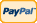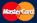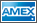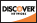Online Store Software Catalog and Price Product Registration Services News About Us Contact Us My Account Español
•Electronics, electrical engineering
•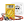Engineering physics
•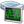Generators
•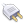Interfaces
•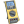Multimeters
•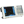Oscilloscopes
•Persona
•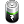Power supplies
•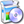Software
•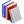Standards
•Thesaurus
•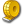Units of measurement
Filter by first letter
A
B
C
D
E
F
G
H
I
J
K
L
M
N
O
P
Q
R
S
T
U
V
W
X
Y
Z
+
-

# Cycle area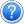Search:

One of the auto measurement types in digital oscilloscopes, relating to a derivative measurement type of amplitude and time, is the definition of an area under one (e.g. the first one) waveform cycle. The cycle area corresponds to the sum of the signal values from the first to the last cycle point, relative to the zero level, multiplied by the time interval between the extreme cycle points. The values which are above zero and measured above the ground potential level give a positive contribution into the area; the values which are below zero and measured below the ground potential – negative. Waveform cycle area is expressed in volt-seconds.

The animated picture below demonstrates a sinusoidal signal waveform with a variable constant component. The first cycle area of the signal is marked in blue. The calculated value of the area under the first waveform cycle is displayed in the measurement results display zone.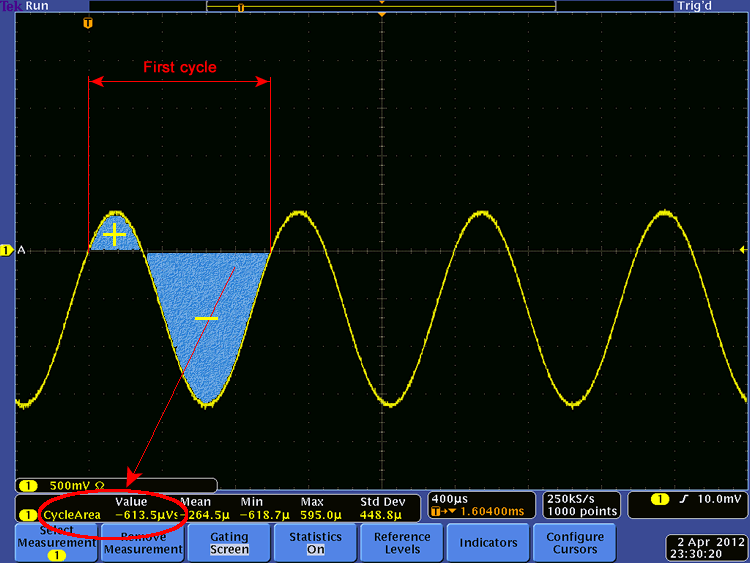Back to the list

Units Converter
• How it Works
• Company News
• Industry News & Events
• Measurement Fun Facts